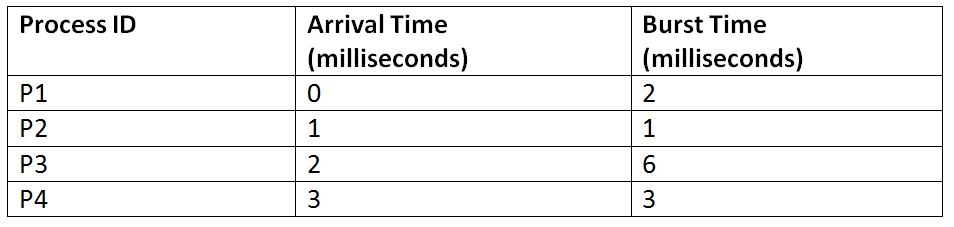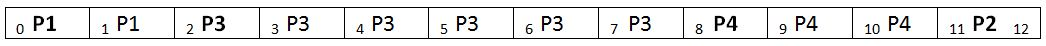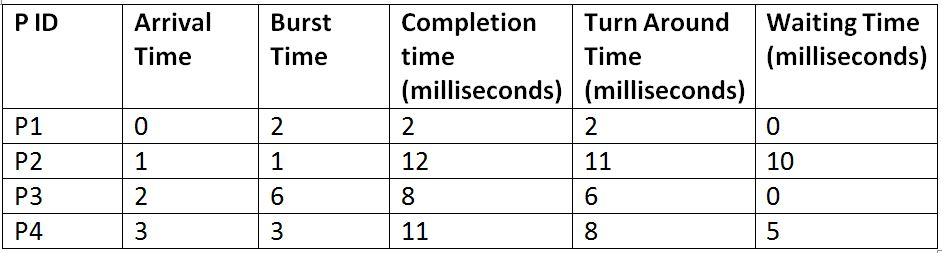# LJF: Longest Job First Scheduling Algorithm

Submitted by Monika Sharma, on June 23, 2019

The LJF, which stands for Longest Job Scheduling Algorithm keeps track of the Burst time of all the available processes at the arrival time itself and then assigns the processor to that process which has the longest burst time. It a type of non-preemptive scheduling algorithm where once a process starts its execution, it cannot be interrupted in between its processing and any other process can be executed only after the assigned process has completed its processing and has been terminated.

The LJF scheduling algorithm is just as the SJF. The only difference is that The SJF scheduling algorithm executes the processes with the shortest burst time first, whereas, in LJF, the processes with the longest burst time are executed first. However, LJF is not considered to be an efficient way of process scheduling because there are many drawbacks of it like the Convoy effect is displayed by it, it has very large average turn-around time and average waiting time. This results in slow processing and decreases the effectiveness of the system.

Let us explain it with the help of an example to understand it better. Suppose there are four processes with process ID's P1, P2, P3, and P4 and they enter into the CPU as follows:So, if the OS follows the FCFS algorithm for scheduling these processes, then they will be executed in the following manner:

Gant Chart:Explanation: As the processes keep arriving, the one with the largest burst time are executed first. And no process can interrupt in the execution of the other process in between.```    Total Turn Around Time = 2 + 11 + 6 + 8
= 27 milliseconds
Average Turn Around Time= Total Turn Around Time / Total No. of Processes
= 27 / 4
= 6.75 milliseconds

Total Waiting Time = 0 + 10 + 0 + 5
= 15 milliseconds
Average Waiting Time = Total Waiting Time / Total No. of Processes
= 15 / 4
= 3.75 milliseconds
```